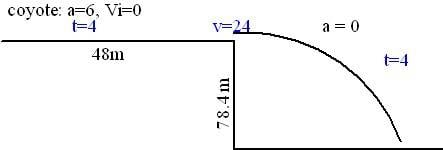# NEED PHYSICS HELP am i doing this correctly?

• terryW16

#### terryW16

Once again the determined coyote is out to get the road runner. He has a new pair of power skates that provide a constant acceleration of 6.0 m/s^2 along the flat top of the mesa he starts off from rest 48.0 m from teh edge of a cliff just as the road runner zips past towards the cliff at constant speed. a) calculate how long it takes the coyote to reach the edge of the cliff. b) what is his speed as he gets there? c) calculate the minimum constant speed of the road runner in order to escape the clutches of the coyote. d) calculate how far from the cliffs edge the coyote lands in the canyon 78.4 m below. His power skates do not provide acceleration when he is air borne. e) what is his velocity on the cactus laden canyon base when he lands there?

## Homework Equations

a) Calculate how long it takes the coyote to reach the edge of the cliff.
V= V0 + at
24.0 m/s= 0 + (6.0 m/s²)t
t= 4.0sec

b) What is his speed just as he gets there?
V²= V0² + 2as
V²= 0 + 2(6.0 m/s²)(48.0m)
V²= 576 m²/s²
V= √576 m/s
V= 24.0 m/s

c) Calculate the minimum constant speed of the roadrunner in order to escape the clutches of the coyote.
Min Speed = 48.0 m/ 4.0 s = 12.0 m/s

d) Calculate how far from the cliff’s edge the coyote lands in the canyon 78.4 m below. His power skates do not provide acceleration when he is air-borne.
Y= y0 + Vy0(t) + (1/2)ay(t)²
Y= y0 + Vy0(t) – (1/2)g(t)²
0= 78.4 m + 0 – (1/2)(9.8 m/s²)t²
0= 78.4 + 0 – 4.9t²
4.9t²= 78.4
t²= 16
t= √16
t= 4.0sec

x= x0 + vx0(t) + (1/2)ax(t)²
x= 0 +(24.0 m/s)(4.0s)+(1/2)(6.0 m/s²)(4.0s)²
x= 144 m

e) What is his velocity on the cactus laden canyon base when he lands there?
Vx= Vx0 + ax(t)
Vx= (24.0 m/s) + (6.0 m/s²)(4.0s)
Vx= 48.0 m/s

Vy= Vy0 + ay(t)
Vy= Vy0 – g(t)
Vy= 0 – (9.8 m/s²)(4.0s)
Vy= -39.2 m/s

V=(vx² +vy²)^1/2 = (48)² + (39.2)²)^1/2 = 61.972 m/s

## The Attempt at a Solution

All correct except the (1/2)(6.0 m/s²)(4.0s)² in part (d) should be omitted because "His power skates do not provide acceleration when he is air borne."
Jolly good work!

...

## Homework Equations

a) Calculate how long it takes the coyote to reach the edge of the cliff.
V= V0 + at
24.0 m/s= 0 + (6.0 m/s²)t
t= 4.0sec
This is the time it takes the coyote to go 24 m/s starting from rest. Why would that be the time to the cliff?

b) What is his speed just as he gets there?
V²= V0² + 2as
V²= 0 + 2(6.0 m/s²)(48.0m)
V²= 576 m²/s²
V= √576 m/s
V= 24.0 m/s
If you do this step before doing part (a), then your answer to part (a) makes sense.
c) Calculate the minimum constant speed of the roadrunner in order to escape the clutches of the coyote.
Min Speed = 48.0 m/ 4.0 s = 12.0 m/s
This looks good.

d) Calculate how far from the cliff’s edge the coyote lands in the canyon 78.4 m below. His power skates do not provide acceleration when he is air-borne.
Y= y0 + Vy0(t) + (1/2)ay(t)²
Y= y0 + Vy0(t) – (1/2)g(t)²
0= 78.4 m + 0 – (1/2)(9.8 m/s²)t²
0= 78.4 + 0 – 4.9t²
4.9t²= 78.4
t²= 16
t= √16
t= 4.0sec
The above is correct for the time to reach the canyon floor.
x= x0 + vx0(t) + (1/2)ax(t)²
x= 0 +(24.0 m/s)(4.0s)+(1/2)(6.0 m/s²)(4.0s)²
x= 144 m
Why use an acceleration of 6.0 m/s2 ??

e) What is his velocity on the cactus laden canyon base when he lands there?
Vx= Vx0 + ax(t)
Vx= (24.0 m/s) + (6.0 m/s²)(4.0s)
Vx= 48.0 m/s
This is also incorrect.

Vy= Vy0 + ay(t)
Vy= Vy0 – g(t)
Vy= 0 – (9.8 m/s²)(4.0s)
Vy= -39.2 m/s
vy looks good.

V=(vx² +vy²)^1/2 = (48)² + (39.2)²)^1/2 = 61.972 m/s
The method here looks good, but vx was incorrect.

It IS just a bit confusing unless you read (b) before (a).
Maybe better to find the time in (a) using d = ½ a⋅t²

Using the d=1.5a*t
48.0=1/2 6.0* t^2
48/(1/2*6.0)= 16
=4.0 ??

and for the Vx= Vx0 + ax(t)
Vx= (24.0 m/s) + (6.0 m/s²)(4.0s)
Vx= 48.0 m/s
what did i do wrong?

and for

x= x0 + vx0(t) + (1/2)ax(t)²
x= 0 +(24.0 m/s)(4.0s)+(1/2)(6.0 m/s²)(4.0s)²
x= 144 m

what should i use instead of the 6.0?
Im pretty frustrated that i feel completely lost on my homeowork today thanks for the help!

What is the acceleration in the horizontal direction when he's airborne ?

Wait, your first solution for (b) was correct!
You can also get it with Vf = Vi + at = 0 + 6*4 = 24.
For (d), the acceleration is zero when airborne:
x= x0 + vx0(t) + (1/2)ax(t)²
x= 0 +(24.0 m/s)(4.0s)+(1/2)(0)(4.0s)²
x= 96 m

You are very good at this, just a tiny bit of confusion about details in the question. Recommend you make a habit of writing down the numbers in the question in a way that makes sense to you at a glance.
I wrote